# RS Aggarwal Class 6 Solutions Chapter 1 Number System Ex 1C

## RS Aggarwal Class 6 Solutions Chapter 1 Number System Ex 1C

These Solutions are part of RS Aggarwal Solutions Class 6. Here we have given RS Aggarwal Solutions Class 6 Chapter 1 Number System Ex 1C

Other Exercises

Question 1.
Solution:
Number of persons in the first year = 13789509
Number of persons in the second year = 12976498
Total number of persons in the two years =13789509 +12976498
= 26766007 Ans.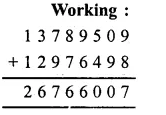Question 2.
Solution:
Number of sugar bags in the first factory = 24809565
Number of sugar bags in the second factory = 18738576
Number of sugar bags in the third factory = 9564568
Total number of sugar bags in the three factories
= 24809565 + 18738576 + 9564568
= 53112709 bags Ans.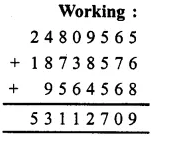Question 3.
Solution:
The given number = 37684955
The number which exceeds the given number by 3615045 will be
= 37684955 + 3615045
= 41300000 Ans.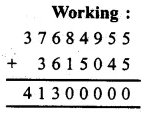Question 4.
Solution:
Number of invalid votes = 13849
Number of persons who did not vote = 25467
Total number of registered votes = 687905 + 495086 + 93756 + 13849 + 25467
= 1316063 Ans.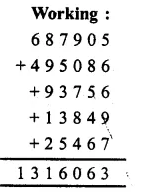Question 5.
Solution:
Number of people who got primary education = 1623546
Number of people who got secondary education = 9768678
Number of people who got higher education = 6837954
Number of illiterate people = 2684536
Number of children below the age of admission = 698781
Total population of the state = 1623546 + 9768678 + 6837954 + 2684536 + 698781
= 21613495 Ans.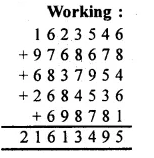Question 6.
Solution:
In the first year, number of cycles produced = 8765435
In the second year, number of cycles produced = 8765435 + 1378689
= 10144124
The number of bicycles produced in the two years = 8765435 + 10144124
= 18909559 Ans.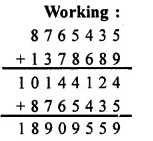Question 7.
Solution:
Sale receipt during first year = Rs. 20956480
Sale receipt during the second year = Rs. 20956480 + Rs. 6709570
= Rs. 27666050
Total sale receipt during the two years = Rs. 20956480 + Rs.27666050
= Rs. 48622530 Ans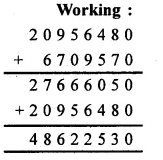Question 8.
Solution:
Total population of a city = 28756304
Number of males = 16987059
Number of females = 28756304 – 16987059
= 11769245 Ans.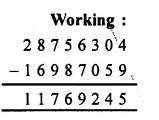Question 9.
Solution:
The number 13246510 is larger than 4658642
= 13246510 – 4658642
= 8587868 Ans.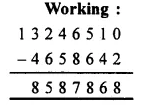Question 10.
Solution:
5643879 is smaller than one crore
= 10000000 – 5643879
= 4356121 Ans.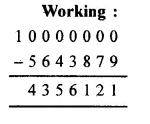Question 11.
Solution:
To, get the required number, we should subtract 2635967 from 11010101
= 11010101 – 2635967
= 8374134 Ans.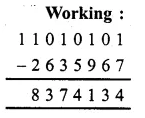Question 12.
Solution:
Sum of two numbers = 10750308
First number = 8967519
Second number = 10750308 – 8967519
= 1782789 Ans.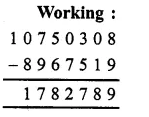Question 13.
Solution:
Total money, a man had = Rs 20000000
Amount spent on buying a school building = Rs. 13607085
Amount left with him
= Rs. 20000000 – Rs. 13607085
= Rs. 6392915 Ans.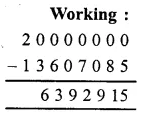Question 14.
Solution:
The total requirement of a society = Rs. 18536000
Amount of fee collection = Rs. 7253840
Amount of loan taken = Rs. 5675450
Amount of donation = Rs. 2937680
Total amount collected = Rs. 7253840 + Rs. 5675450 + Rs. 2937680
= Rs. 15866970
Short amount
= Rs. 18536000 – Rs. 15866970
= Rs. 2669030 Ans.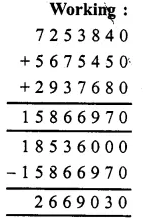Question 15.
Solution:
Total amount a man had = Rs. 10672540
Amount given to his wife = Rs. 4836980
Amount given to his son = Rs 3964790
Total amount given to wife and son = Rs. 4836980 + Rs 3964790
= Rs. 8801770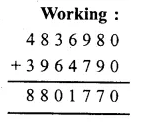Balance amount given to his daughter
= Rs. 10672540 – Rs. 8801770
= Rs. 1870770 Ans.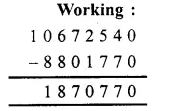Question 16.
Solution:
Cost of one chair = Rs. 1485
Cost of 469 chairs = Rs. 1485 x 469
= Rs. 696465 Ans.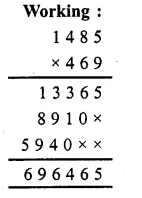Question 17.
Solution:
Collection from one student = Rs. 625
Collection from 1786 students = Rs. 1786 x 625
= Rs. 1116250 Ans.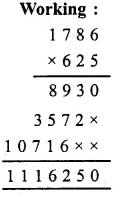Question 18.
Solution:
Number of screws produced in one day = 6985
Number of screws produced in 358 days = 6985 x 358
= 2500630 Ans.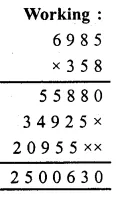Question 19.
Solution:
Number of months in one year = 12
Number of months in 13, years = 12 x 13
= 156
months Saving in one month = Rs. 8756
Saving in 156 months = Rs. 8756 x 156
= Rs. 1365936 Ans.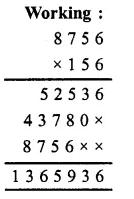Question 20.
Solution:
Cost of 1 scooter = Rs. 36725
Cost of 487 scooters = Rs. 36725 x 487
= Rs. 17885075 Ans.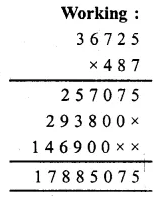Question 21.
Solution:
Distance covered in 1 hour = 1485 km
Distance covered in 72 hours = 1485 x 72 km
= 106920 km Ans.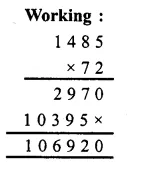Question 22.
Solution:
Product of two numbers = 13421408
First number = 364
Second number = 13421408 ÷ 364
= 36872 Ans.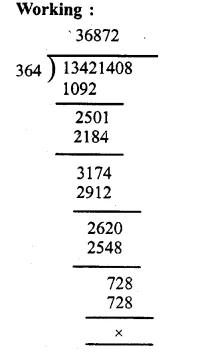Question 23.
Solution:
Cost of 36 flats = Rs. 68251500
Cost of one flat
= Rs. 68251500 ÷ 36
= Rs. 1895875 Ans.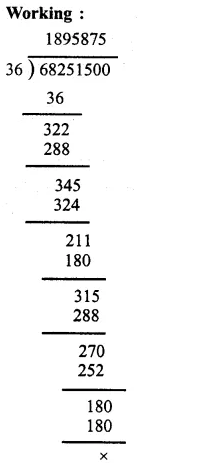Question 24.
Solution:
Mass of cylinder with gas = 30 kg 250 g and mass of empty cylinder = 14 kg 480 g
Mass of gas = 30 kg, 250 g – 14 kg, 480 g
= 15 kg, 770 g Ans.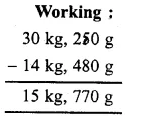Question 25.
Solution:
Total length of cloth = 5 m
Length of piece cut off = 2 m 85 cm
Length of remaining piece of cloth = 5 m – 2 m 85 cm
= 2 m 15 cm Ans.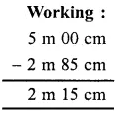Question 26.
Solution:
Cloth required for 1 shirt = 2 m 75 cm
Cloth required for 16 shirts = 2 m 75 cm x 16
= 44 m Ans.

Question 27.
Solution:
Total length of cloth for 8 trousers = 14 m 80 cm
Length of cloth for 1 trouser = 14 m 80 cm ÷ 8
= 1 m 85 cm Ans.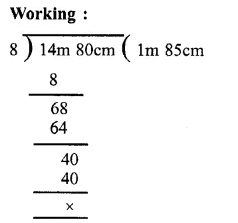Question 28.
Solution:
Mass of 1 brick = 2 kg 750 g
Total mass of 14 bricks = 2 kg 750 g x 14
= 38 kg 500 g Ans.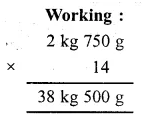Question 29.
Solution:
Total mass of 8 packets = 10 kg 600 g
Mass of one packet = 10 kg 600 ÷ 8
= 1 kg 325 g Ans.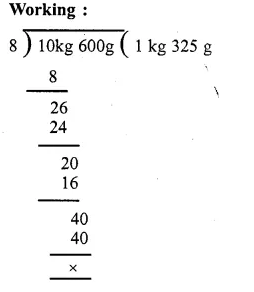Question 30.
Solution:
Total length of rope = 10 m
No of pieces = 8
Length of each piece = 10 m ÷ 8
= 1 m 25 cm Ans.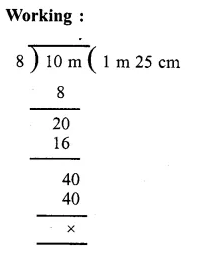Hope given RS Aggarwal Solutions Class 6 Chapter 1 Number System Ex 1C are helpful to complete your math homework.

If you have any doubts, please comment below. Learn Insta try to provide online math tutoring for you.